Uniwersytet w Białymstoku - Centralny System Uwierzytelniania Nie jesteś zalogowany | zaloguj się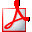Drukuj sylabus

# Probability Calculus II

## Informacje ogólne

 Kod przedmiotu: 360-MS1-3RP2a Kod Erasmus / ISCED: 11.102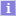/ (0541) MatematykaNazwa przedmiotu: Probability Calculus II Jednostka: Wydział Matematyki Grupy: Punkty ECTS i inne: 4.00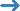zobacz reguły punktacji Język prowadzenia: angielski Rodzaj przedmiotu: obowiązkowe Założenia (opisowo): (tylko po angielsku) Student should know basic methods from mathematical analysis and Probability Calculus I. Skrócony opis: (tylko po angielsku) Course profile: academic Form of study: stationary Academic discipline: Mathematics, field of study in science: mathematics Prerequisities: Mathematical Analysis, Probablility Calculus I lecture 30 h. exercise class 30 h. ECTS credits: 4 Pełny opis: (tylko po angielsku) Course is devoted to probabilistic methods in theory and applications. After course students should know basic methods and theorems in random variables, conditional expected value, characteristic functions, limit theorems and stochastic processes. Topics: 1. Random variables and their distributions 2. Conditional expected value 3. Characteristic functions for random variables 4. Sequences of random variables and their distributions 5. Central limit theorem 6. Elements of stochastic processes 7. Markov chains 8. Wiener stochastic process and Ito integral Verification methods: lectures, exercises, consultations, studying literature, home works, discussions in groups. Balance of student workload: attending lectures15x2h = 30h attending exercise classes 15x2h = 30h preparation for classes 7x3h = 21h completing notes after exercises and lectures 7x2h = 14h consultations 12x1h = 12h the final examination: preparation.and take 12h + 3h = 15h control works: repeating the material and preparation 3x4h = 12h Quantitative description Direct interaction with the teacher: 75 h., 3 ECTS Literatura: (tylko po angielsku) 1. H. Tucker A graduate Course in Probablility, Dover Publ. 2014 2. T. Brzeźniak, T. Zastawniak Basic Stochastic Processes. A course through exercises, Springer 1999 3. M. Capiński, E. Kopp, Measure, Integral and Probability, Springer 2004 4. P. Billingsley Probability and Measure, Willey 2012 Efekty uczenia się: (tylko po angielsku) Learning outcomes: Student knows probability distributions of basic random variables, such as normal distribution, gamma distribution, Poisson distribution etc. Student knows theorems and properties of convergence of sequences of random variables. Student understand the notion of conditional expected value and uses it in practise Student knows characteristic functions for basic probability distributions and their properties Student knows Central Limit Theorem and uses it in practise Student understand the notion of Markov chain, knows classification of states in Markov chain and calculates probabilities of states. Student understand the notion of Wiener process and calculates Ito integral. Metody i kryteria oceniania: (tylko po angielsku) The overall form of credit for the course: final exam

## Zajęcia w cyklu "Rok akademicki 2022/23" (jeszcze nie rozpoczęty)

 Okres: 2022-10-01 - 2023-06-30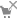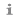Wybrany podział planu: ten tydzień cykl przedmiotuzobacz plan zajęć Typ zajęć: Ćwiczenia, 30 godzinwięcej informacji Wykład, 30 godzinwięcej informacji Koordynatorzy: Tomasz Czyżycki, Aneta Sliżewska Prowadzący grup: (brak danych) Lista studentów: (nie masz dostępu) Zaliczenie: Egzamin Skrócony opis: (tylko po angielsku) Course profile: academic Form of study: stationary Academic discipline: Mathematics, field of study in science: mathematics Prerequisities: Mathematical Analysis, Probablility Calculus I lecture 30 h. exercise class 30 h. ECTS credits: 4 Pełny opis: (tylko po angielsku) Course is devoted to probabilistic methods in theory and applications. After course students should know basic methods and theorems in random variables, conditional expected value, characteristic functions, limit theorems and stochastic processes. Topics: 1. Random variables and their distributions 2. Conditional expected value 3. Characteristic functions for random variables 4. Sequences of random variables and their distributions 5. Central limit theorem 6. Elements of stochastic processes 7. Markov chains 8. Wiener stochastic process and Ito integral Verification methods: lectures, exercises, consultations, studying literature, home works, discussions in groups. Balance of student workload: attending lectures15x2h = 30h attending exercise classes 15x2h = 30h preparation for classes 7x3h = 21h completing notes after exercises and lectures 7x2h = 14h consultations 12x1h = 12h the final examination: preparation.and take 12h + 3h = 15h control works: repeating the material and preparation 3x4h = 12h Quantitative description Direct interaction with the teacher: 75 h., 3 ECTS Literatura: (tylko po angielsku) 1. H. Tucker A graduate Course in Probablility, Dover Publ. 2014 2. T. Brzeźniak, T. Zastawniak Basic Stochastic Processes. A course through exercises, Springer 1999 3. M. Capiński, E. Kopp, Measure, Integral and Probability, Springer 2004 4. P. Billingsley Probability and Measure, Willey 2012
Opisy przedmiotów w USOS i USOSweb są chronione prawem autorskim.
Właścicielem praw autorskich jest Uniwersytet w Białymstoku.